You are here: Info Library > Electricity > Logic Gates
Info Library
Options

# Electricity > Logic Gates

A logic gate is an electrical system which process signals that represent TRUE or FALSE. Usually TRUE is represented by a positive supply voltage (+V) and FALSE by 0V. At any given moment, each terminal is in one of the Binary conditions, that is, either a "1" or a "0" which are represented by different voltages, eg. "1" can be +5V and "0" 0V.

The following examples are the most commonly taught gates.

## NOT GATE

The output Q is not true when the input A is true and vice versa. A NOT gate is sometimes called an inverter or a logical inverter.

Symbol IEC symbol Input A Output Q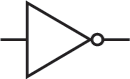1 0
 0 1

## NAND GATE

A NAND gate is an AND gate with an inverted output. The inversion is denoted by the circle on the output.

Symbol IEC symbol Input A Input B Output Q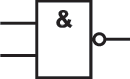0 0 1 1
 0 1 0 1
 1 1 1 0

## NOR GATE

The NOR gate is a combination of an OR gate and an inverter. If both inputs are false, the output is true. Otherwise the output is false.

Symbol IEC symbol Input A Input B Output Q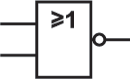0 0 1 1
 0 1 0 1
 1 0 0 0

## XNOR GATE

The XNOR gate is the same as the XOR gate but with an inverted output. The output is true if the inputs are the same and false if the inputs are different.

Symbol IEC symbol Input A Input B Output Q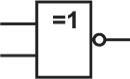0 0 1 1
 0 1 0 1
 1 0 0 1

## XOR GATE

The output is true if either but not both inputs are true. The output is false if both inputs are false or if both are true.

Symbol IEC symbol Input A Input B Output Q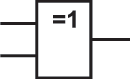0 0 1 1
 0 1 0 1
 0 1 1 0

## AND GATE

The output is true if BOTH inputs are true. An AND gate can have two or more inputs. The output is true of all inputs are true.

Symbol IEC symbol Input A Input B Output Q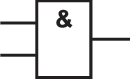0 0 1 1
 0 1 0 1
 0 0 0 1

## OR GATE

The output is true if either inputs (or both) are true.

Symbol IEC symbol Input A Input B Output Q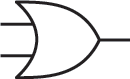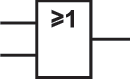0 0 1 1
 0 1 0 1
 0 1 1 1